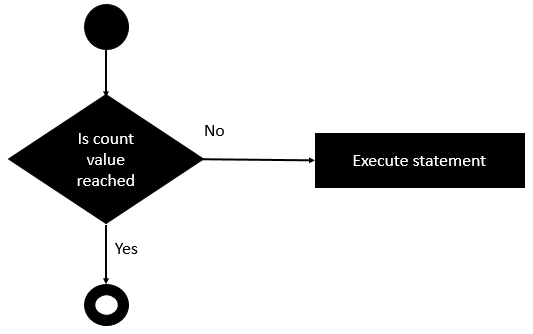# Rexx - do-while Loop

The do-while statement is used to simulate the simple while loop which is present in other programming languages.

## Syntax

The syntax of the do-while statement is as follows −

```do while (condition)
statement #1
statement #2
...
end
```

The while statement is executed by first evaluating the condition expression (a Boolean value), and if the result is true, then the statements in the while loop are executed. The process is repeated starting from the evaluation of the condition in the while statement. This loop continues until the condition evaluates to false. When the condition is false, the loop terminates. The program logic then continues with the statement immediately following the while statement.

## Flow Diagram

The following diagram shows the diagrammatic explanation of this loop.The key point to note is that the code block runs till the condition in the do loop evaluates to true. As soon as the condition evaluates to false, the do loop exits.

The following program is an example of a do-while loop statement.

## Example

```/* Main program */
j = 1

do while(j <= 10)
say j
j = j + 1
end
```

The following key points need to be noted about the above program.

• We are defining a recursive function called do while which would simulate the implementation of our while loop.

• We are initializing the variable j to a value of 1. This value will be incremented in our do-while loop.

• For each value of j, the do-while loop evaluates whether the value of j is less than or equal to 10. If so, it displays the value of j and increments the value of j accordingly.

The output of the above code will be −

```1
2
3
4
5
6
7
8
9
10
```
rexx_loops.htm